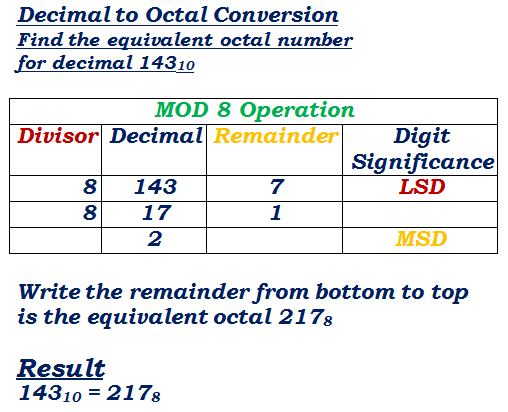# Java Program To Convert Decimal To Octal | Vice Versa

Java program to convert decimal to octal vice versa octal to decimal using standard values, recursion, array, and vice versa. Here are the basic steps just to let you know the conversion from decimal to octal in math. If you have a clear idea about the conversion then skip the below tutorial and check out the programming – Here is the

• What is octal?

An octal is a computer-based system with base eight. The digits in math are 0, 1, 2, 3, 4, 5, 6, 7. The value to the base eight represents as single 8 or Zero or 108.

• What is Decimal?

A decimal number system is a term with base 10. It’s most commonly used number system.  The decimal number system consists of 10 digits from 0, 1, 2, 3, 4, 5, 6, 7, 8, 9.

How to convert from decimal to octal?source

For Example, take one number e.g. 1792 when it is divided by 8, you will get quotient ‘ 224 ‘ remainder ‘ 0 ‘.Followed by 224 /8 you will get Q = 28, R = 0 if you continue like that the result in octal as = 3400. Hope you get it. Here is the Java code to print conversion.

1. Using Static Method

• Here is the code in static format #with sample outputs.

Output:

2. Using Recursion Method

Here is the code using recursion method with sample output code

Output:

3. Basic Program

Output:

4. Convert Octal To Decimal: Vice versa

Here is the code from to convert from octal to decimal.

Output:

x

## X Star Pattern Java Program – Patterns

Java program to print X star pattern program – We have written the below print/draw ...